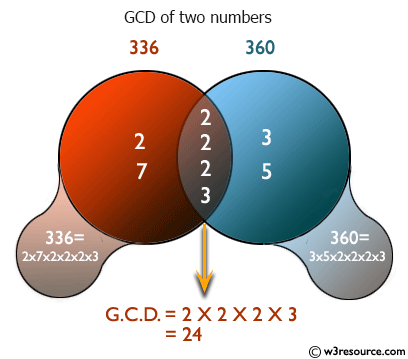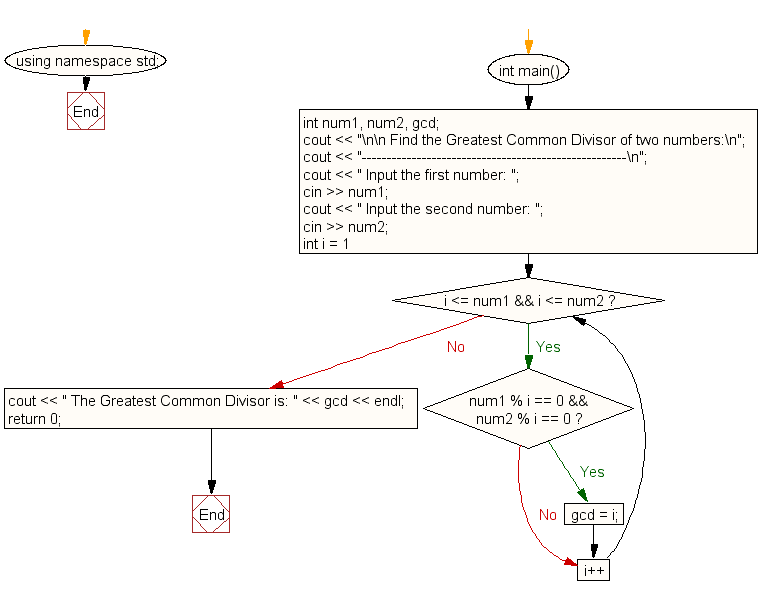﻿ C++ : Find the Greatest Common Divisor (GCD) of two numbers

# C++ Exercises: Find the Greatest Common Divisor (GCD) of two numbers

## C++ For Loop: Exercise-9 with Solution

Write a program in C++ to find the Greatest Common Divisor (GCD) of two numbers.

Pictorial Presentation:Sample Solution :-

C++ Code :

``````#include <iostream>
using namespace std;
int main()
{
int num1, num2, gcd;
cout << "\n\n Find the Greatest Common Divisor of two numbers:\n";
cout << "-----------------------------------------------------\n";
cout << " Input the first number: ";
cin >> num1;
cout << " Input the second number: ";
cin >> num2;

for (int i = 1; i <= num1 && i <= num2; i++)
{
if (num1 % i == 0 && num2 % i == 0)
{
gcd = i;
}
}
cout << " The Greatest Common Divisor is: " << gcd << endl;
return 0;
}
``````

Sample Output:

``` Find the Greatest Common Divisor of two numbers:
-----------------------------------------------------
Input the first number: 25
Input the second number: 15
The Greatest Common Divisor is: 5
```

Flowchart:C++ Code Editor: